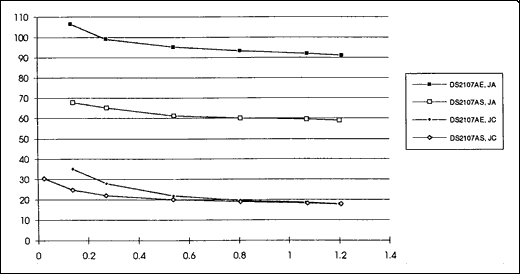# DS2107A Thermal Considerations

### Abstract

This application note describes the techniques used to determine the ΘJA and ΘJC for the DS2107A, and how to calculate power dissipation.

The DS2107A is used in SCSI systems to provide active termination for 9 signal lines. In the typical 8 bit-wide data configuration (A cable), two DS2107A's are required to fully terminate the bus (9 control lines + 8 data lines + 1 parity line). In the 16-bit wide data configuration (P cable), three DS2107A's are required to fully terminate the bus (9 control lines + 16 data lines + 2 parity lines). The two packages available are DS2107AS, 16-pin SOIC, and DS2107AE, 20-pin TSSOP (Thin Shrink Small Outline Package).

Semiconductor die temperature is a key characteristic in semiconductor operation and long-term reliability. Thermal resistance, theta (Θ), is used in determining the heat dissipation capability of a semiconductor package. A low Θ value corresponds to better heat conduction. Junction, case and ambient temperatures are important because these are the areas of power dissipation. ΘJA and ΘJC are the junction-to-ambient and junction-to-case thermal resistances respectively. The die temperature, TDIE, can be calculated as follows:Where PDISSIPATED is the power dissipated by the semiconductor. An example calculation is shown in Appendix A.

ΘJA and ΘJC were measured on the DS2107AS and DS2107AE using the well known VBE variation of a forward biased diode as a measuring element. This method is preferred for this type of measurement due to the linear dependence of the forward biased diode voltage on temperature and the small amount of power dissipated in the diode to measure junction temperature (~2mW). The diode VBE was measured over temperature to calibrate the die temperature readings. Next, various resistor combinations of the DS2107A were grounded and allowed to run for 10 minutes to insure stability of the die temperature for the given power level. Finally, the diode VBE was measured and referenced to the calibration plot to determine the die temperature. The ΘJA and ΘJC were then calculated from the known ambient and case temperatures. Results of the testing are shown in Table 1. The values are the averages measured at 1 Watt.

Table 1.

 ΘJA ΘJC DS2107AS 60°C/W 19°C/W DS2107AE 93°C/W 19°C/W

Graph 1 shows the results of the measured ΘJA and ΘJC versus the power dissipated. Since the die temperature is more affected by the power dissipation as the power is increased, the most important part of the graph is to the right.

Use of heat sinks in board design improves the thermal characteristics of the DS2107A. The TSSOP package has a custom leadframe connecting the die directly to the heat sink ground pins and all pads are double bonded. This gives the DS2107AE the best thermal performance in the industry for the TSSOP package.Graph 1. ΘJA and ΘJC (°C/W) vs. Power Dissipation (W) DS2107AS, DS2107AE.

### APPENDIX A

Typical or nominal power dissipation can be calculated by using the following five variables:

 RTERM Termination Resistance TERMPWR TERMination PoWeR Voltage VREF Voltage Regulator Output Voltage VSL Voltage on a SCSI signal line when in the zero state %DC Percent duty cycle

The formula to calculate the power in Watts dissipated by the DS2107A is:

((VREF - VSL) / RTERM) × 9 × TERMPWR × %DC

For example, the following calculations represents the power dissipation under normal conditions.

 Parameter Nominal Value RTERM 110Ω TERMPWR 5.0V VREF 2.85V VSL 0.5V %DC 30%

The PDISSIPATED under normal conditions would be:

((2.85 - 0.5) / 110) × 9 × 5.0 × 30% = 0.288 Watts